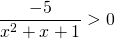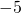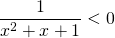# Solution assignment 03 Inequalities with fractions

### Assignment 3

Solve:### Solution

Negative numbers are less easy to handle and therefore we divide both left- and right-hand side by. Then we get (note that dividing both sides by a negative number changes the direction of the inequality sign):The graph of the quadratic function in the denominator is an 'opens up' parabola which does not have intersection points with the-axis (because the discriminant is negative). So the graph is positive for all values of. There is no value offor which the fraction will be negative.

0